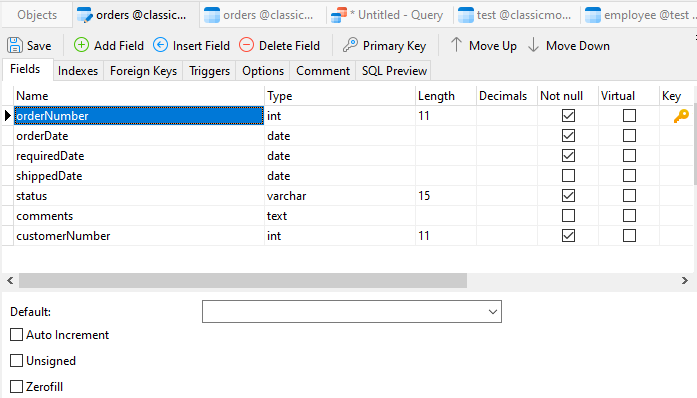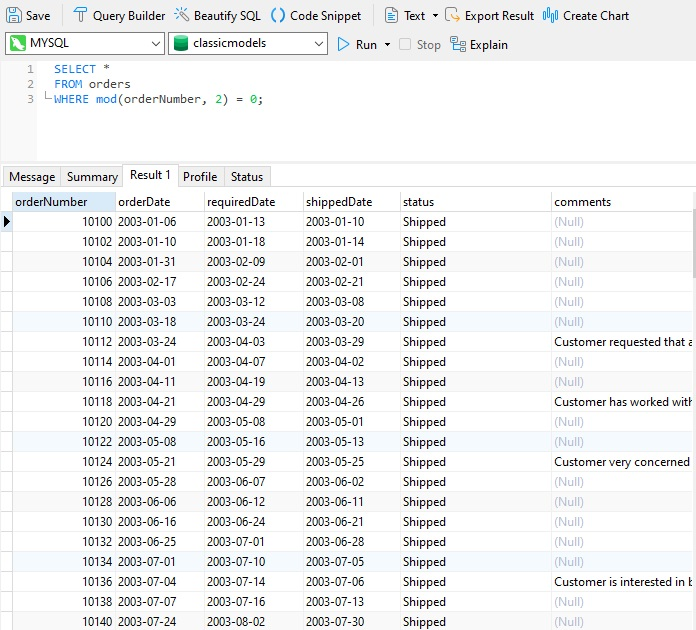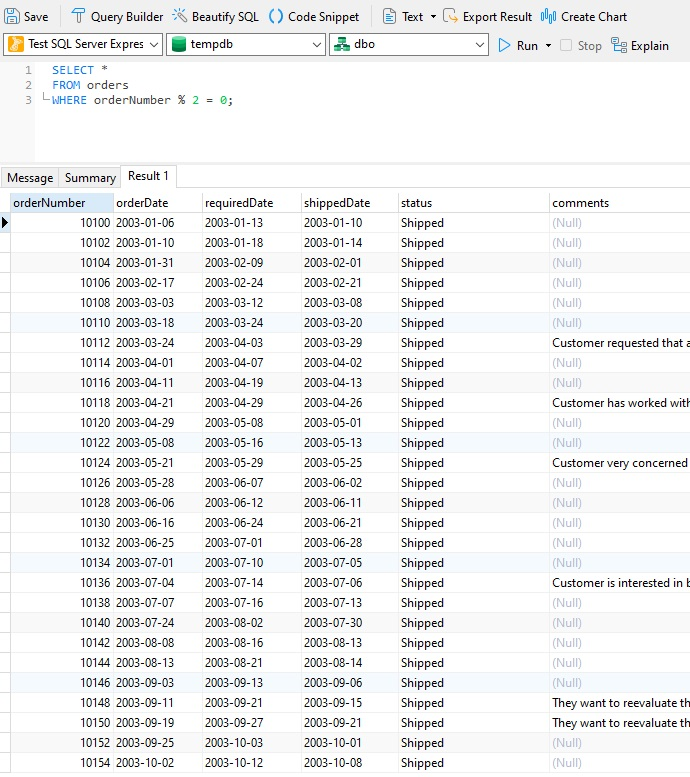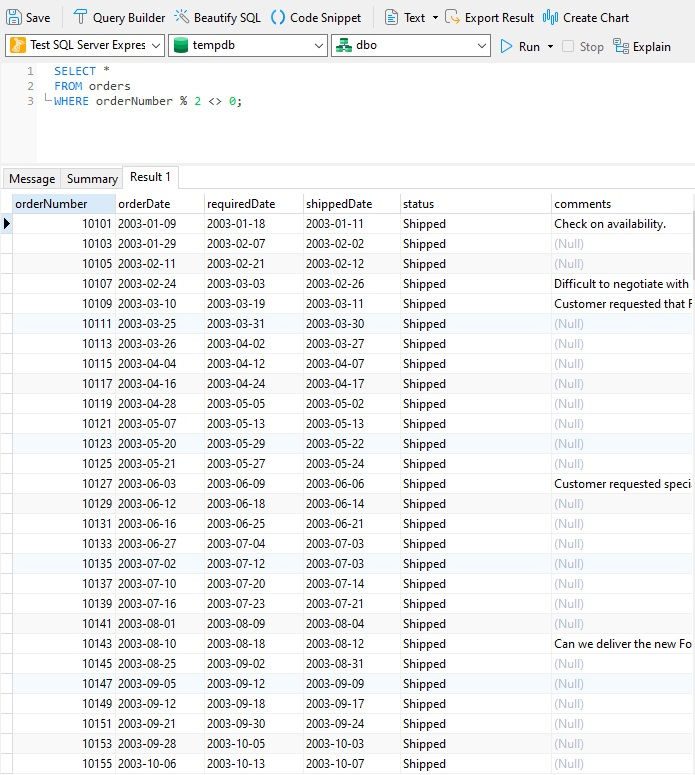# Navicat Blog

Selecting Odd or Even Numbered Rows From a Table Jul 8, 2022 by Robert Gravelle

Having to select only odd or even rows from a table sounds like something that you'd never have to do, that is until you do. A quick Google search confirms that it's something that is done often enough, but, with few database practitioners knowing how, they invariably turn to online database communities in search of answers. As a reader of this blog, you can save yourself the trouble of scouring database forums for a solution, as we'll set the record straight right here today.

# Picking a Suitable Target Column

Before we can speak of "even or odd rows" we have to order the rows by the column whose data we're interested in splitting. Ideally, its data should be numeric, unique, and sorted in ascending order. Hence, auto-increment columns like those of a primary key make perfect candidates. Otherwise, you may need to write a subquery with an ORDER BY clause and then select from it.

As an example, let's open the orders table of the classicmodels sample database in Navicat Premium 16's Table Designer. We can see that its PK (the orderNumber column) is not auto-incrementing, as evidenced by the unchecked "Auto Increment" checkbox:However, opening the table in Grid View shows that orderNumber values are clearly sorted in ascending order:Hence, we can write a query directly against the table.

# Solutions By Database

The simplest way to find the records with odd or even values is to check the remainder when we divide the column value by 2. A remainder of 0 indicates an even number, while an odd number points to an odd number. However, like so many database tasks, how you go about determining the remainder depends on what type of database you're working with.

In PostgreSQL, MySQL, and Oracle, we can use the MOD() function to check the remainder:

Here's the general query syntax to find rows where a specified column has even values:

```SELECT *
FROM table_name
WHERE mod(column_name,2) = 0;
```

This syntax will find rows where our target column has odd values:

```SELECT *
FROM table_name
WHERE mod(column_name,2) <> 0;
```

SQL Server does not have a MOD function. Instead, it provides the % modulus operator.

Here's the general query syntax to find rows where a specified column has even values:

```SELECT *
FROM table_name
where column_name % 2 = 0;
```

This syntax will find rows where our target column has odd values:

```SELECT *
FROM table_name
where column_name % 2 <> 0;
```

# Some Examples

Let's give each of the above statements a try against the orders table of the classicmodels sample database, first in SQL, then in SQL Server.

First, we'll retrieve even rows:Next, we'll fetch odd rows only:As mentioned previously, SQL Server does not have a MOD function, so well employ the % modulus operator instead.

Even rows:Odd rows:# Conclusion

This blog presented an easy way to retrieve odd or even numbered rows from various databases by checking the remainder after dividing the target column value by 2 - a solution that is both simple and effective.

Blog Archives
Share
• Key Topics• Products• Account• Partners